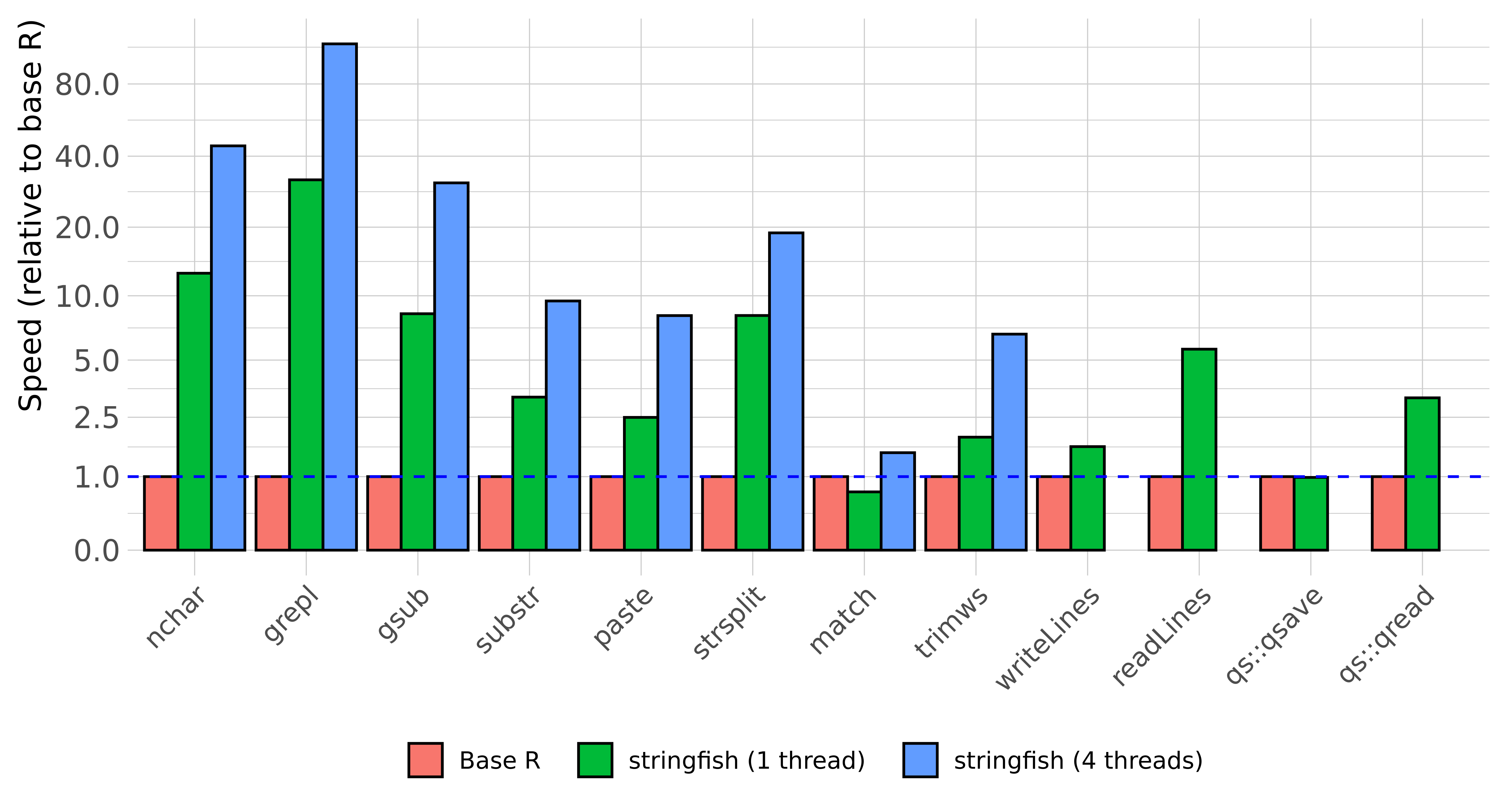# stringfish

stringfish is a framework for performing string and sequence operations using the ALTREP system to speed up the comptuation of common string operations.

The ultimate goal of the package is to unify ALTREP string implementations under a common framework.

The ALTREP system (new as of R 3.5.0) allows package developers to represent R objects using their own custom memory layout, completely invisible to the user. stringfish represents string data as a simple C++/STL vector, which is very fast andlightweight.

Using normal R functions to process string data (e.g. substr, gsub, paste, etc.) causes “materialization” of ALTREP vectors to normal R data, which can be a slow process. Therefore, in order to take full advantage of the ALTREP framework, string processing functions need to be re-written to be ALTREP aware. This package hopes to fulfill that purpose.

## Installation:

install.packages("stringfish", type="source", configure.args="--with-simd=AVX2")

## Benchmark

The simplest way to show the utility of the ALTREP framework is through a quick benchmark comparing stringfish and base R.Yes you are reading the graph correctly: some functions in stringfish are more than an order of magnitude faster than vectorized base R operations (and even faster with some build in multithreading). On large text datasets, this can turn minutes of computation into seconds.

## Currently implemented functions

A list of implemented stringfish function and analogous base R function:

• sf_iconv (iconv)
• sf_nchar (nchar)
• sf_substr (substr)
• sf_paste (paste0)
• sf_collapse (paste0)
• sf_readLines (readLines)
• sf_writeLines (writeLines)
• sf_grepl (grepl)
• sf_gsub (gsub)
• sf_toupper (toupper)
• sf_tolower (tolower)
• sf_starts (startsWith)
• sf_ends (endsWith)
• sf_trim (trimws)
• sf_split (strsplit)
• sf_match (match for strings only)
• sf_compare/sf_equals (==, ALTREP-aware string equality)

Utility functions:

• sf_vector – creates a new and empty stringfish vector
• sf_assign – assign strings into a stringfish vector in place (like x[i] <- "mystring")
• sf_convert/convert_to_sf – converts a character vector to a stringfish vector
• get_string_type – determines string type (whether ALTREP or normal)
• materialize – converts any ALTREP object into a normal R object
• random_strings – creates a random strings as either a stringfish or normal R vector
• string_identical – like identical for strings but also requires identical encoding (i.e. latin1 and UTF-8 strings will not match)

In addition, many R operations in base R and other packages are already ALTREP-aware (i.e. they don’t cause materialization). Functions that subset or index into string vectors generally do not materialize.

• sample
• head
• tail
• [ – e.g. x[20:30]
• dplyr::filter – e.g. dplyr::filter(df, sf_starts("a"))
• Etc.

stringfish functions are not intended to exactly replicate their base R analogues. One difference is that subject parameters are always the first argument, which is easier to use with pipes (%>%). E.g., gsub(pattern, replacement, subject) becomes sf_gsub(subject, pattern, replacement).

## Extensibility

stringfish as a framework is intended to be easily extensible. Stringfish vectors can be worked into Rcpp scripts or even into other packages (see the qs package for an example).

Below is a detailed Rcpp script that creates a function to alternate upper and lower case of strings.

// [[Rcpp::plugins(cpp11)]]
// [[Rcpp::depends(stringfish)]]
#include <Rcpp.h>
#include "sf_external.h"
using namespace Rcpp;

// [[Rcpp::export]]
SEXP sf_alternate_case(SEXP x) {
// Iterate through a character vector using the RStringIndexer class
// If the input vector x is a stringfish character vector it will do so without materialization
RStringIndexer r(x);
size_t len = r.size();

// Create an output stringfish vector
// Like all R objects, it must be protected from garbage collection
SEXP output = PROTECT(sf_vector(len));

// Obtain a reference to the underlying output data
sf_vec_data & output_data = sf_vec_data_ref(output);

// You can use range based for loop via an iterator class that returns RStringIndexer::rstring_info e
// rstring info is a struct containing const char * ptr (null terminated), int len, and cetype_t enc
// a NA string is represented by a nullptr
// Alternatively, access the data via the function r.getCharLenCE(i)
size_t i = 0;
for(auto e : r) {
// check if string is NA and go to next if it is
if(e.ptr == nullptr) {
i++; // increment output index
continue;
}
// create a temporary output string and process the results
std::string temp(e.len, '\0');
bool case_switch = false;
for(int j=0; j<e.len; j++) {
if((e.ptr[j] >= 65) & (e.ptr[j] <= 90)) { // char j is upper case
if((case_switch = !case_switch)) { // check if we should convert to lower case
temp[j] = e.ptr[j] + 32;
continue;
}
} else if((e.ptr[j] >= 97) & (e.ptr[j] <= 122)) { // char j is lower case
if(!(case_switch = !case_switch)) { // check if we should convert to upper case
temp[j] = e.ptr[j] - 32;
continue;
}
} else if(e.ptr[j] == 32) {
case_switch = false;
}
temp[j] = e.ptr[j];
}

// Create a new vector element sfstring and insert the processed string into the stringfish vector
// sfstring has three constructors, 1) taking a std::string and encoding,
// 2) a char pointer and encoding, or 3) a CHARSXP object (e.g. sfstring(NA_STRING))
output_data[i] = sfstring(temp, e.enc);
i++; // increment output index
}
// Finally, call unprotect and return result
UNPROTECT(1);
return output;
}

Example function call:

sf_alternate_case("hello world")
 "hElLo wOrLd"